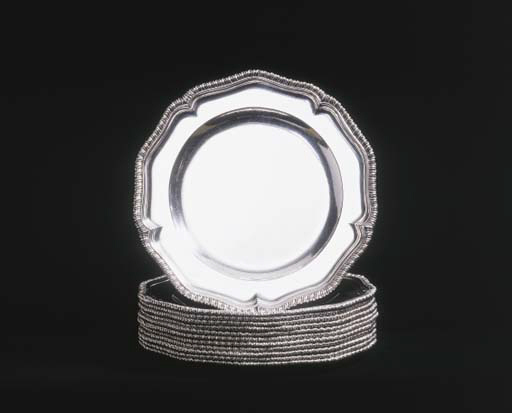263A SET OF TWELVE GEORGE II SILVER DINNER PLATES OF HEAVY GAUGE

# MAKER'S MARK OF JOHN LUFF, LONDON, 1743

Details
A SET OF TWELVE GEORGE II SILVER DINNER PLATES OF HEAVY GAUGE
Maker's mark of John Luff, London, 1743
Each shaped circular with gadrooned border, marked on reverses, also engraved with inventory number and scratchweights: No. 2 22=10=2 No. 8 21=8=2 No. 22 20=14=0 No. 35 12=6=0 No. 38 21=12=0 No. 44 20=17=2 No. 47 22=10=0 No. 51 20=19=0 No. 56 22=8=0 No. 63 20=16=2 No. 64 22=0=0 No. 67 21=12=2
9½in. (24cm.); 253oz. (7876gr.) (12)

## Lot Essay

SUPP IMAGE TEXT:
Detail of reverse, lot 263

View All